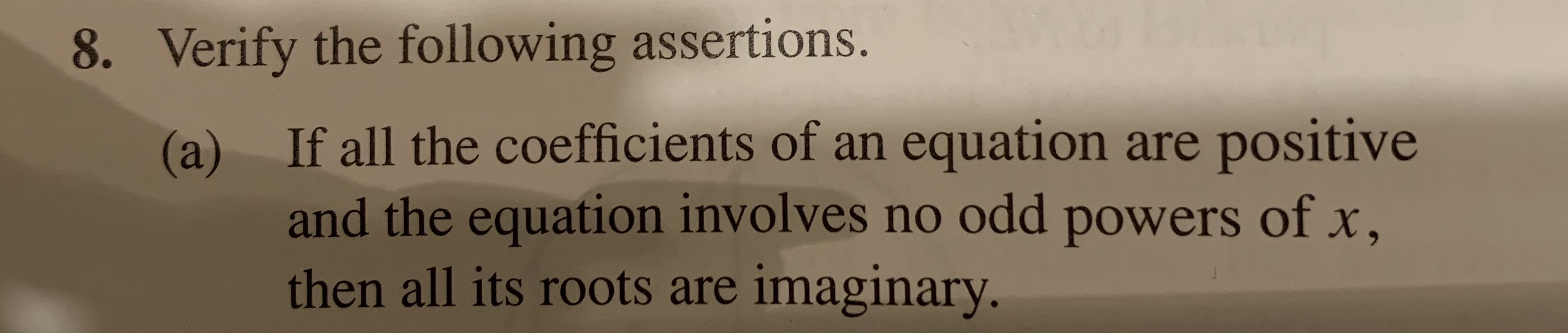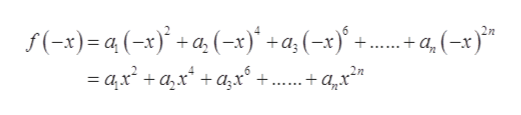# 8. Verify the following assertions.If all the coefficients of an equation are positive(a)and the equation involves no odd powers of x,then all its roots are imaginary.

Question
32 views

#8a using Descartes's rule of signhelp_outlineImage Transcriptionclose8. Verify the following assertions. If all the coefficients of an equation are positive (a) and the equation involves no odd powers of x, then all its roots are imaginary. fullscreen
check_circle

Step 1

Prove in the following that if all the coefficients of an equation are positive and the equation involves no odd powers of x, then all its roots are imaginary.

Step 2

Take an equation,

Step 3

There is no sign change between all the terms. Therefore, there is no positiv...help_outlineImage Transcriptionclosef(-x)=a(-x)a (-x)+a (-x)+ 6 22 + q (-x) - ax + аx* + ад+. fullscreen

### Want to see the full answer?

See Solution

#### Want to see this answer and more?

Solutions are written by subject experts who are available 24/7. Questions are typically answered within 1 hour.*

See Solution
*Response times may vary by subject and question.
Tagged in

### Math